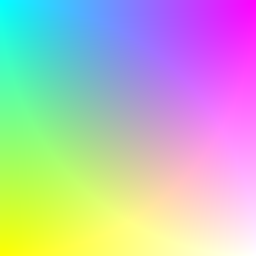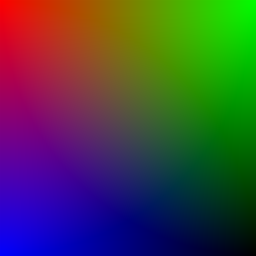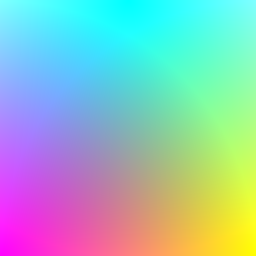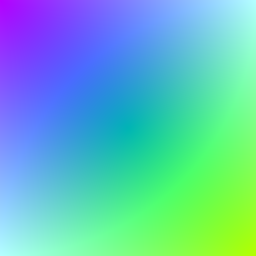example processing for-loop
``````void setup() {
size(256, 256);
noSmooth();
}

void draw() {
for(int y = 0; y < height; y++){
for(int x = 0; x < width; x++){
float distanceFromTopLeft = dist(x, y, 0, 0);
float distanceFromTopRight = dist(x, y, width, 0);
float distanceFromBottomLeft = dist(x, y, 0, height);

stroke(distanceFromTopLeft, distanceFromTopRight, distanceFromBottomLeft);
point(x, y);
}
}
}
``````
Code Editor

See the Pen by Happy Coding (@KevinWorkman) on CodePen.

This code uses nested `for` loops to loop over every pixel in the window. For each pixel, it creates a color based on that pixel’s distance from the corners, and then draws the pixel in that color. This creates a corner gradient.All of the magic happens on these lines:

``````float distanceFromTopLeft = dist(x, y, 0, 0);
float distanceFromTopRight = dist(x, y, width, 0);
float distanceFromBottomLeft = dist(x, y, 0, height);

stroke(distanceFromTopLeft, distanceFromTopRight, distanceFromBottomLeft);
``````

This code uses the `dist()` function to get the distance between each pixel and the top-left, top-right, and bottom-left corners. It then passes those distances into the `stroke()` function, which sets its red, green, and blue components.

Try playing with the parameters passed into the `stroke()` function to come up with different colors, or move the points around to create different types of gradients.## Tweak Ideas

• Instead of using the corners, use points that bounce around the screen.
• Pass in a random number as one of the parameters to the `stroke()` function.